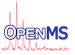OpenMS  2.8.0
OpenMS::FastLowessSmoothing Namespace Reference

LOWESS (locally weighted scatterplot smoothing). More...

## Functions

int lowess (const std::vector< double > &x, const std::vector< double > &y, double f, int nsteps, double delta, std::vector< double > &result)
Computes a lowess smoothing fit on the input vectors. More...

int lowess (const std::vector< double > &x, const std::vector< double > &y, std::vector< double > &result)
Computes a lowess smoothing fit on the input vectors with the recommended values. More...

## Detailed Description

LOWESS (locally weighted scatterplot smoothing).

A non-parametric smoothing technique that fits a simple linear regression model to localized subsets of the data, point by point. This is often used for retention time alignments.

The implementation here is optimized for speed and many datapoints. Note that it performs a linear fit, it does not implement quadratic fits. It is based on the initial FORTRAN code by W. S. Cleveland published at NETLIB.

Note that this should work best for for large datasets with mostly linear behavior. For small datasets with non-linear behavior, use the LowessSmoothing class.

## ◆ lowess() [1/2]

 int OpenMS::FastLowessSmoothing::lowess ( const std::vector< double > & x, const std::vector< double > & y, double f, int nsteps, double delta, std::vector< double > & result )

Computes a lowess smoothing fit on the input vectors.

This is a fast implementation of a lowess fit that is based on the original Fortran code by W. S. Cleveland and it uses some optimizations.

Parameters
 x The input vector in the first dimension y The input vector in the second dimension f Fraction of datapoints to use for each local regression (the span, recommended value: 2/3) nsteps The number of robustifying iterations (recommended value: 3) delta nonnegative parameter which may be used to save computations (recommended value: 0.01 * range of x) result Result of fit
Precondition
The size of the vectors x and y needs to be equal
The vector needs to have at least 2 elements
The vector x needs to be sorted
The f value needs to be between 0 and 1
The nsteps parameter needs to be zero or larger
The delta parameter needs to be zero or larger

The delta parameter allows the algorithm to not perform the regression at every data point, as it assumes that points that are close to each other will have the same regression parameters. A linear interpolation is used to fill in the skipped points, larger values lead to increased speed up.

The f parameter allows the caller to influence the smoothness. A larger values will increase smoothness (recommended value: 2/3) It is the fraction of points used to compute each fitted value. Choosing F in the range .2 to .8 usually results in a good fit

The nsteps parameter controls how many iterations are performed in the robust fit (setting it to zero turns off the robust fit and the nonrobust fit is returned). A value of 2 or 3 should be sufficient for most purposes.

## ◆ lowess() [2/2]

 int OpenMS::FastLowessSmoothing::lowess ( const std::vector< double > & x, const std::vector< double > & y, std::vector< double > & result )

Computes a lowess smoothing fit on the input vectors with the recommended values.

Parameters
 x The input vector in the first dimension y The input vector in the second dimension result Result of fit
Precondition
The size of the vectors x and y needs to be equal
The vector needs to have at least 2 elements
The vector x needs to be sorted